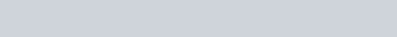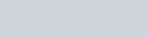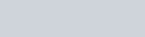In this video I introduce you to the general solutions for the second order linear differential equation

•2nd Order Linear Differential Equations - Auxillary Equation : Introduction : ExamSolutions - youtube Video

Solving equations where b2 – 4ac > 0

In this video I give a worked example of the general solution for the second order linear differential equation which has real and different roots.

Solve the following:

1.2nd Order Linear Differential Equations - Auxillary Equation : Real Different Roots : ExamSolutions - youtube Video

Solving equations where b2 – 4ac = 0

In this video I give a worked example of the general solution for the second order linear differential equation which has real and different roots.

Solve the following:

1.2nd Order Linear Differential Equations - Auxillary Equation : Equal Roots : ExamSolutions - youtube Video

Solving equations where b2 – 4ac < 0

In this video I give a worked example of the general solution for the second order linear differential equation which has imaginary roots.

Solve the following:

1.2nd Order Linear Differential Equations - Auxillary Eqn Imaginary Roots : ExamSolutions - youtube Video